Volume Patterns for Pyramids

In nearly all of the important formulas for measuring objects, the different dimensions appear either in the exponents or the coefficients. For volumes of cones and pyramids, the dimensions show up in two different ways.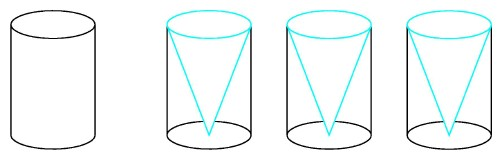The contents of three conical cups exactly fill a cylinder of the same radius and height.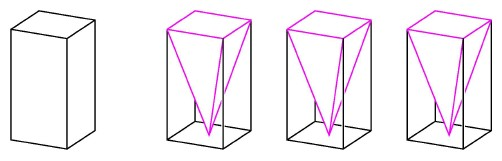The contents of three pyramids with rectangular bases exactly fill a prism of the same base and height.

We do not know which ancient artisan first filled a cone three times from the water in a cylinder and thereby observed that the volume of a cylinder is three times the volume of a cone with like base and height. This relationship was later recognized to hold in a wide range of figures: a prism with rectangular sides and square or triangular base has three times the volume of a pyramid with like base and height. Once we observe this relationship, we can express it in formula: the volume of a cylinder or prism is the area of the base multiplied by the height, and the volume of a cone or pyramid is one-third the volume of the corresponding cylinder or prism.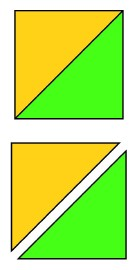Two congruent right triangles meet along a diagonal of a square.

The relationship between volumes of prisms and volumes of pyramids is analogous to the relationship between areas of rectangles and areas of triangles in the plane. A diagonal line divides a square into two congruent isosceles right triangles. More generally, the area of a rectangle is the length of the base multiplied by the height, and the area of a triangle is one-half the length of the base multiplied by the height. The pattern lies in the denominatior of the fraction. If we consider a four-dimensional analogue of a pyramid, then its four-dimensional volume should be one-fourth the volume of its three-dimensional base multiplied by its height in a fourth direction.

Mathematcians are not content merely to observe a pattern: they want to find an argument that shows why the same pattern will always occur. Experiments with water-filled pyramids do not provide a proof of the volume formula in three dimensions. Fortunately we can establish this relationship by decomposing a cube into three congruent pyramids. Just as a square is decomposed into two congruent triangles which meet along a diagonal line, a cube can be decomposed into three identical pieces which meet along a diagonal of the cube. Each of the pieces is a square-based pyramid, with its top point directly over one corner of the square base. It follows that the volume of each piece is one-third the volume of the cube. From the relationships in the second and third dimensions, the pattern is already clear. We cannot construct a real model to illustrate the analogous relationship in the fourth dimension, but we can still proceed to formulate hvpotheses. We expect that a four-dimensional hypercube can be decomposed into four congruent off-center four-dimensional pyramids meeting along the longest diagonal of the hypercube. In general the n-dimensioiial cube should be decomposable into n congruent off-center n-dimensional pyramids, each with an (n-1)-dimensional cube as its base.

The pattern appears both in the exponents and in the denominators of the formulas for area and volume of an off-center pyramid. The area of an isosceles triangle with side length mis m2/2. The volume of an off-center pyramid obtained from a cube of side length m is m3/3. The pattern predicts that the four-dimensional volume of an off-center four-dimensional pyramid obtained from a hvpercube of side length m is m4/4 . The analogous formula for the n-dimensional volume of an off-center n-dimensional pyramid is mn/n.Three congruent pyramids meet along a diagonal of a cube.

The analogy between the decomposition of the square in the plane and the cube in space gave us a way to find the volume formula for an off-center pyramid with a square base. We would like to prove a formula for a more general class of pyramids with rectangular bases, but unfortunately the analogy between a rectangular region in the plane and a rectangular prism in space is not a perfect one. A square is divided into two congruent triangles by a diagonal, and so is a rectangular region. But even though a cube can be divided into three congruent pieces meeting along a diagonal, it is usually not possible to decompose a rectangular prism into three congruent pieces meeting along a diagonal. However, it is still possible to divide the rectangular prism into three noncongruent pieces of equal volume, and this result relies on a more sophisticated phenomenon, namely the effect of a scale transformation along a single direction.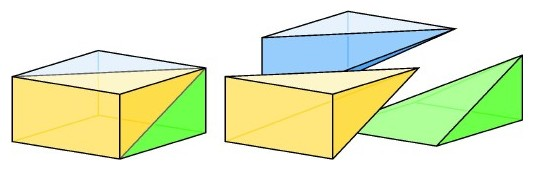Three noncongruent pyramids with equal volume meet along a diagonal of a rectangular prism.

We can approximate the volume of a pyramid by stacking thick rectangular cards parallel to the base. If we double the thickness of each card in the stack, then the base stays the same while the height doubles and the weight of the stack (and therefore its volume) also doubles. If we keep the width and the thickness of each card the same and double the length, then the volume also doubles. Doubling any single dimension causes the volume to double, and in general multiplying a single dimension by any number will multiply the entire volume bv that same number.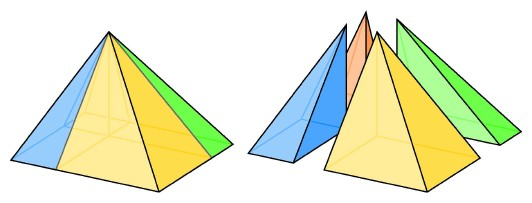A square-based pyramid is decomposed into four rectangle-based pyramids having their top points over one corner.

This procedure enables us to obtain the volume of any pyramid of rectangular base that has its top point directly over a corner of the rectangle. We begin with a cube, having three times the, volume of an off-center pyramid contained within it. We double the length of one of the edges of the cube to form a rectangular prism, silnultaneously forming an off-center pyramid with the same rectangular base. As we double the length of the edge of the prism, its volume doubles. But all the slabs approximating the volume of the off-center pyramid also double, so the pyramid still has a volume one-third the volume of the prism. The basic volume relationship still holds.

What if the top point of the pyramid is located not over the corner but over some other point of the rectangle? We can their divide the pyramid up into four pyramids with the top point over one corner, and the volume of each of these is one-third the area of the base multiplied by their common height. Adding all of these contributions together shows that in general the volume of a pyramid with a rectangular base is one-third the volume of the rectangular prism with the same base and the same height.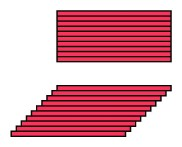Thin strips that fill a rectangle approximate the area of a parallelogram.

It is also possible to deduce this result by applying Cavalieri's principle for shear transformations, which again uses thick slices to approximate areas and volumes: we can fill a parallelogram with the same set of rods that fills a rectangle of the same base and height, and we can fill a slanted box with the same pack of cards that fill a straight box. An off-center pyramid can be approximated with the same collection of square cards that approximate a centered one.

The formulas for volumes of various kinds of square-based pyramids can be modified to give formulas for pyramids formed by cutting off a corner of a cube. The dimension of the space again appears in the denominator of the formula, but in a new way. Cutting off one corner of a square produces a triangle with half the area. Cutting off one corner of a cube produces a pyramid with a triangular base. We can obtain this same triangular pyramid by decomposing a square-based pyramid into four pieces. If we approximate the square-based pyramid by a stack of square slabs, then the triangular pyramid is approximated by a stack of triangular slabs each with half the volume. It follows that the volume of the triangular pyramid is half the volume of the square-based pyramid, or one-third the volume of the triangle-based prism, therefore one-sixth the volume of the cube. The four-dimensional volume of a corner hyperpyramid of a hypercube will be one-fourth the volume of the triangular pyramid which is its three-dimensional base, namely 1/24 the four-dimensional volume of the hypercube. In general the n-dimensional volume of a corner figure of an n-cube will be 1/n!, where n! stands for "n factorial," obtained by, multiplying together the first n numbers.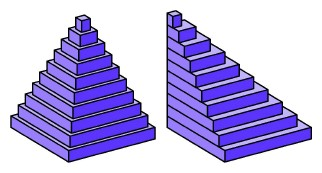The same thin slabs approximate the volume of both a centered square-based pyramid and a pyramid with the same base and its top over one corner.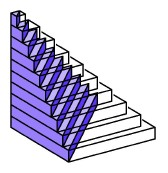Halving the slabs of a square-based pyramid approximates a triangle-based pyramid.